# Making caterpillar plots for random effects in SPSS

For one of my classes for PhD students (in seminar research and analysis), I talk about the distinction between random effect models and fixed effect models for a week.

One of my favorite plots to go with random effect models is called a caterpillar plot. So typically folks just stop at reporting the variance of the random intercepts and slopes when they estimate these models. But you not only get the global variance estimates, but can also get an estimate (and standard error) for each higher level variable. So if I have 100 people, and I do a random intercept for those 100 people, I can say “Joe B’s random intercept is 0.5, and Jane Doe’s random intercept is -0.2” etc.

So this is halfway in between confirmatory data analysis (we used a model to get those estimates) but is often useful for further understanding the model and seeing if you should add anything else. E.g. if you see the random intercepts have a high correlation with some other piece of person information, that information should be incorporated into the model. It is also useful to spot outliers. And if you have spatial data mapping the random intercepts should be something you do.

SPSS recently made it easier to make these types of plot (as of V25), so I am going to give an example. In my class, I give code examples in R, Stata, and SPSS whenever I can, so this link contains code for all three programs. I will be using data from my dissertation, with crime on street segments in DC, nested within regular grid cells (used to approximate neighborhoods).

## SPSS Code

So first data prep, I define where my data is using FILE HANDLE, read in the csv file of the data, compute a new variable (the sum of both detritus and physical infrastructure 311 calls). Then finally I declare that the FishID variable (my grid cells neighborhoods) is a nominal level variable. SPSS needs that defined correctly for later models.

``````*************************************************************.
FILE HANDLE data /NAME = "??????Your Path Here!!!!!!!!!!!".

*Importing the CSV file into SPSS.
GET DATA  /TYPE=TXT
/FILE="data\DC_Crime_withAreas.csv"
/ENCODING='UTF8'
/DELCASE=LINE
/DELIMITERS=","
/QUALIFIER='"'
/ARRANGEMENT=DELIMITED
/FIRSTCASE=2
/DATATYPEMIN PERCENTAGE=95.0
/VARIABLES=
MarID AUTO
XMeters AUTO
YMeters AUTO
FishID AUTO
XMetFish AUTO
YMetFish AUTO
TotalArea AUTO
WaterArea AUTO
AreaMinWat AUTO
TotalLic AUTO
TotalCrime AUTO
CFS1 AUTO
CFS2 AUTO
CFS1Neigh AUTO
CFS2Neigh AUTO
/MAP.
CACHE.
EXECUTE.
DATASET NAME CrimeDC.
DATASET ACTIVATE CrimeDC.

*Compute a new variable, total number of 311 calls for service.
COMPUTE CFS = CFS1 + CFS2.
EXECUTE.

VARIABLE LEVEL FishID (NOMINAL).
*************************************************************.``````

Now onto the good stuff, estimating our model. Here we are looking at the fixed effects of bars and 311 calls on crime on street segments, but also estimating a random intercept for each grid cell. As of V25, SPSS lets you specify an option to print the solution for the random statements, which we can capture in a new SPSS dataset using the OMS command.

So first we declare our new dataset to dump the results in, `Catter`. Then we specify an OMS command to capture the random effect estimates, and then estimate our negative binomial model. I swear SPSS did not use to be like this, but now you need to end the OMS command before you putz with that dataset.

``````*************************************************************.
DATASET DECLARE Catter.

OMS
/SELECT TABLES
/IF SUBTYPES='Empirical Best Linear Unbiased Predictions'
/DESTINATION FORMAT=SAV OUTFILE='Catter' VIEWER=YES
/TAG='RandTable'.

*SOLUTION option only as of V25.
GENLINMIXED
/FIELDS TARGET=TotalCrime
/TARGET_OPTIONS DISTRIBUTION=NEGATIVE_BINOMIAL
/FIXED EFFECTS=TotalLic CFS
/RANDOM USE_INTERCEPT=TRUE SUBJECTS=FishID SOLUTION=TRUE
/SAVE PREDICTED_VALUES(PredRanEff).

OMSEND TAG='RandTable'.
EXECUTE.
DATASET ACTIVATE Catter.
*************************************************************.``````

And now we can navigate over to the saved table and make our caterpillar plot. Because we have over 500 areas, I sort the results and don’t display the X axis. But this lets you see the overall distribution and spot any outliers.

``````*************************************************************.
*Lets make a caterpillar plot.
FORMATS Prediction Std.Error LowerBound UpperBound (F4.2).
SORT CASES BY Prediction (D).

GGRAPH
/GRAPHDATASET NAME="graphdataset" VARIABLES=Var1 Prediction LowerBound UpperBound
/GRAPHSPEC SOURCE=INLINE
/FRAME INNER=YES.
BEGIN GPL
SOURCE: s=userSource(id("graphdataset"))
DATA: Var1=col(source(s), name("Var1"), unit.category())
DATA: Prediction=col(source(s), name("Prediction"))
DATA: LowerBound=col(source(s), name("LowerBound"))
DATA: UpperBound=col(source(s), name("UpperBound"))
SCALE: cat(dim(1), sort.data())
GUIDE: axis(dim(1), null())
GUIDE: axis(dim(2), label("BLUP"))
SCALE: linear(dim(2), include(0))
ELEMENT: point(position(Var1*Prediction), color.interior(color.black), size(size."1"))
END GPL.
*************************************************************.``````

And here is my resulting plot.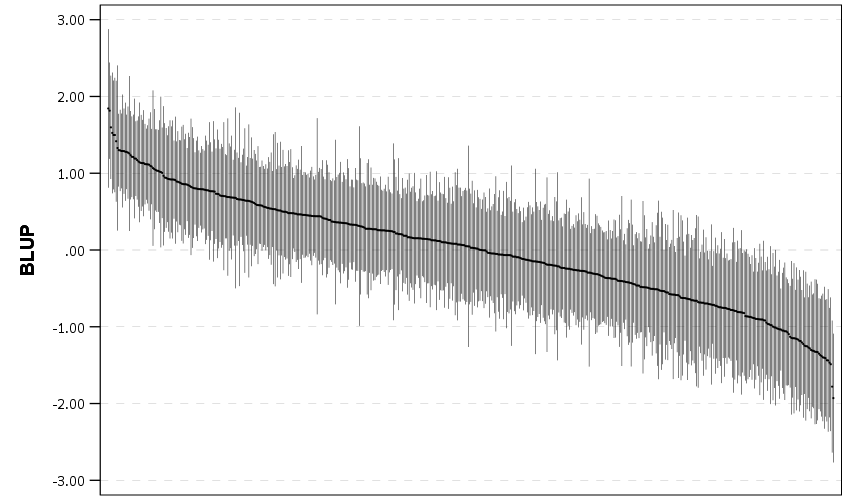And I show in the linked code some examples for not only random intercepts, but you can do the same thing for random slopes. Here is an example doing a model where I let the TotalLic effect (the number of alcohol licenses on the street segment) vary by neighborhood grid cell. (The flat 0 estimates and consistent standard errors are grid cells with 0 licenses in the entire area.)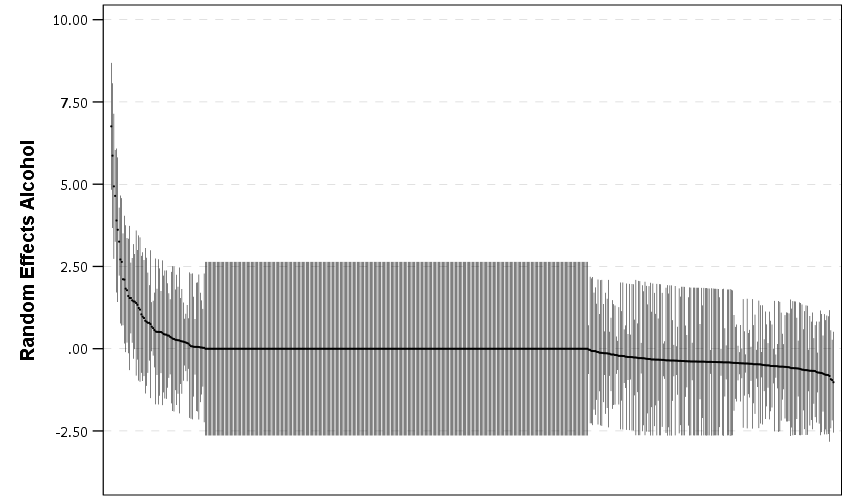The way to interpret these estimates are as follows. The fixed effect part of the regression equation here is: `0.247 + 0.766*Licenses`. That alcohol license effect though varies across the study area, some places have a random slope of +2, so the equation could then be thought of as `0.247 + (0.766 + 2)*Licenses` (ignoring the random intercept part). So the effect of bars in that area is much larger. Also there are places with negative effects, so the effects of bars in those places are smaller. You can do the same type of thought experiments simply with the reported variance components, but I find the caterpillar plots to be a really good visual to show what those random effects actually mean.

For other really good multilevel modelling resources, check out the Centre for Multilevel Modelling, and Germán Rodríguez’s online notes. Eventually I will get around to uploading my seminar class notes and code snippets, but in the mean time if you see a week and would like my code examples, always feel free to email.

# Making a hexbin map in ggplot

In a recent working paper I made a hexbin map all in R. (Gio did most of the hard work of data munging and modeling though!) Figured I would detail the process here for some notes. Hexagon binning is purportedly better than regular squares (to avoid artifacts of runs in discretized data). But the reason I use them in this circumstance is mostly just an aesthetic preference.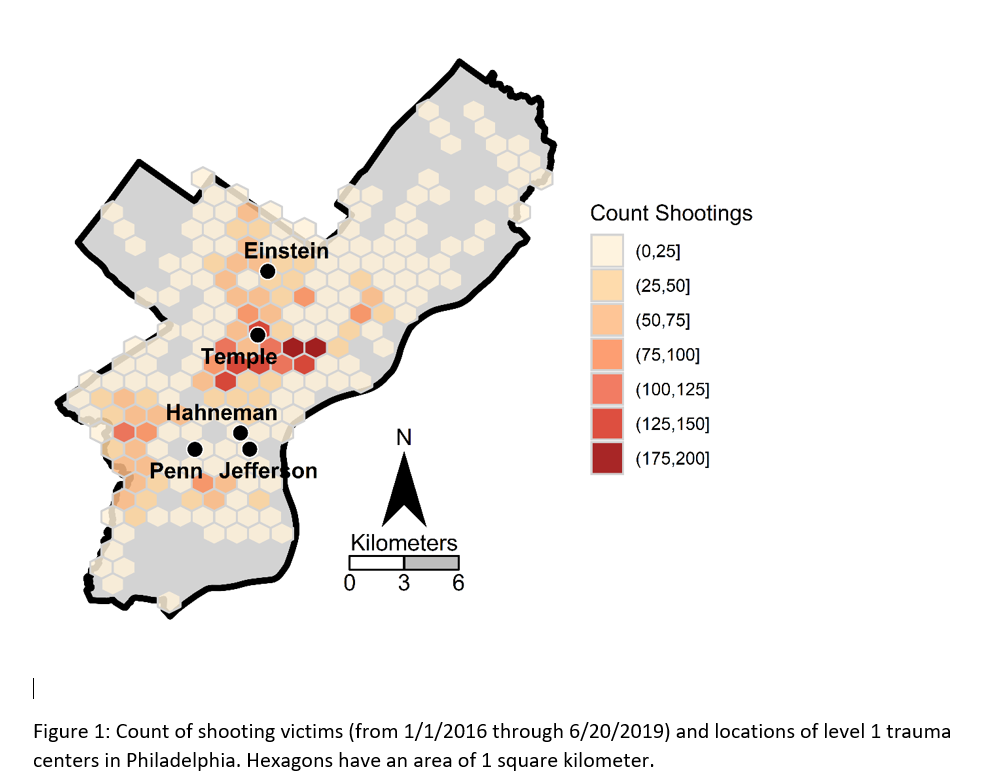Two tricky parts to this: 1) making the north arrow and scale bar, and 2) figuring out the dimensions to make regular hexagons. As an illustration I use the shooting victim data from Philly (see the working paper for all the details) full data and code to replicate here. I will walk through a bit of it though.

## Data Prep

First to start out, I just use these three libraries, and set the working directory to where my data is.

``````library(ggplot2)
library(rgdal)
library(proj4)
setwd('C:\\Users\\axw161530\\Dropbox\\Documents\\BLOG\\HexagonMap_ggplot\\Analysis')``````

Now I read in the Philly shooting data, and then an outline of the city that is projected. Note I read in the shapefile data using `rgdal`, which imports the projection info. I need that to be able to convert the latitude/longitude spherical coordinates in the shooting data to a local projection. (Unless you are making a webmap, you pretty much always want to use some type of local projection, and not spherical coordinates.)

``````#Read in the shooting data
#Get rid of missing
shoot <- shoot[!is.na(shoot\$lng),c('lng','lat')]
#Project the Shooting data
phill_pj <- proj4string(PhilBound)
XYMeters <- proj4::project(as.matrix(shoot[,c('lng','lat')]), proj=phill_pj)
shoot\$x <- XYMeters[,1]
shoot\$y <- XYMeters[,2]``````

## Making a Basemap

It is a bit of work to make a nice basemap in R and ggplot, but once that upfront work is done then it is really easy to make more maps. To start, the `GISTools` package has a set of functions to get a north arrow and scale bar, but I have had trouble with them. The `ggsn` package imports the north arrow as a bitmap instead of vector, and I also had a difficult time with its scale bar function. (I have not figured out the `cartography` package either, I can’t keep up with all the mapping stuff in R!) So long story short, this is my solution to adding a north arrow and scale bar, but I admit better solutions probably exist.

So basically I just build my own polygons and labels to add into the map where I want. Code is motivated based on the functions in `GISTools`.

``````#creating north arrow and scale bar, motivation from GISTools package
arrow_data <- function(xb, yb, len) {
s <- len
arrow.x = c(0,0.5,1,0.5,0) - 0.5
arrow.y = c(0,1.7  ,0,0.5,0)
adata <- data.frame(aX = xb + arrow.x * s, aY = yb + arrow.y * s)
}

scale_data <- function(llx,lly,len,height){
box1 <- data.frame(x = c(llx,llx+len,llx+len,llx,llx),
y = c(lly,lly,lly+height,lly+height,lly))
box2 <- data.frame(x = c(llx-len,llx,llx,llx-len,llx-len),
y = c(lly,lly,lly+height,lly+height,lly))
return(list(box1,box2))
}

x_cent <- 830000
len_bar <- 3000
offset_scaleNum <- 64300
arrow <- arrow_data(xb=x_cent,yb=67300,len=2500)
scale_bxs <- scale_data(llx=x_cent,lly=65000,len=len_bar,height=750)

lab_data <- data.frame(x=c(x_cent, x_cent-len_bar, x_cent, x_cent+len_bar, x_cent),
y=c( 72300, offset_scaleNum, offset_scaleNum, offset_scaleNum, 66500),
lab=c("N","0","3","6","Kilometers"))``````

This is about the best I have been able to automate the creation of the north arrow and scale bar polygons, while still having flexibility where to place the labels. But now we have all of the ingredients necessary to make our basemap. Make sure to use `coord_fixed()` for maps! Also for background maps I typically like making the outline thicker, and then have borders for smaller polygons lighter and thinner to create a hierarchy. (If you don’t want the background map to have any color, use `fill=NA`.)

``````base_map <- ggplot() +
geom_polygon(data=PhilBound,size=1.5,color='black', fill='darkgrey', aes(x=long,y=lat)) +
geom_polygon(data=arrow, fill='black', aes(x=aX, y=aY)) +
geom_polygon(data=scale_bxs[], fill='grey', color='black', aes(x=x, y = y)) +
geom_polygon(data=scale_bxs[], fill='white', color='black', aes(x=x, y = y)) +
geom_text(data=lab_data, size=4, aes(x=x,y=y,label=lab)) +
coord_fixed() + theme_void()

#Check it out
base_map``````

This is what it looks like on my windows machine in RStudio — it ends up looking alittle different when I export the figure straight to PNG though. Will get to that in a minute.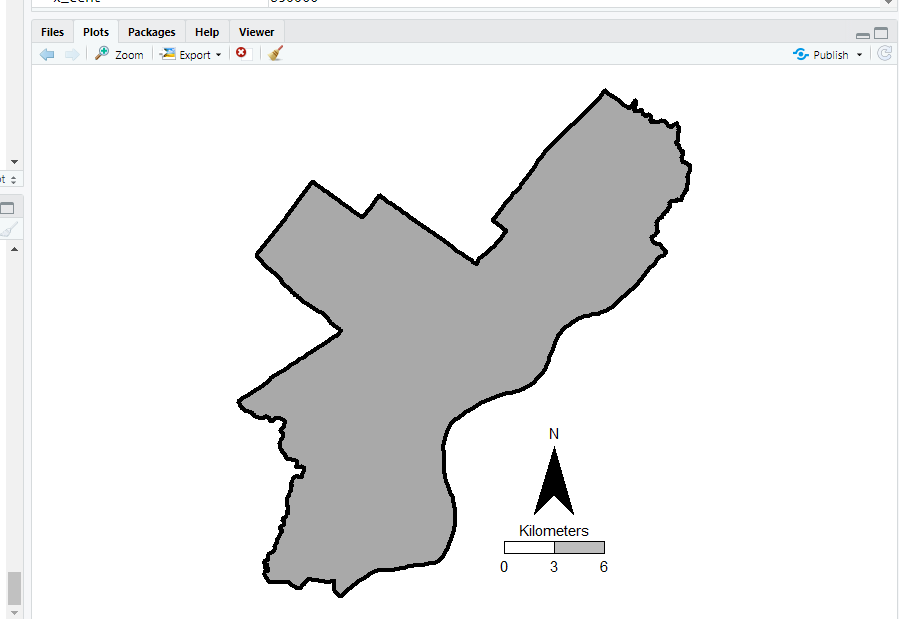## Making a hexagon map

Now you have your basemap you can superimpose whatever other data you want. Here I wanted to visualize the spatial distribution of shootings in Philly. One option is a kernel density map. I tend to like aggregated count maps though better for an overview, since I don’t care so much for drilling down and identifying very specific hot spots. And the counts are easier to understand than densities.

In `geom_hex` you can supply a vertical and horizontal parameter to control the size of the hexagon — supplying the same for each does not create a regular hexagon though. The way the hexagon is oriented in `geom_hex` the vertical parameter is vertex to vertex, whereas the horizontal parameter is side to side.

Here are three helper functions. First, `wd_hex` gives you a horizontal width length given the vertical parameter. So if you wanted your hexagon to be vertex to vertex to be 1000 meters (so a side is 500 meters), `wd_hex(1000)` returns just over 866. Second, if for your map you wanted to convert the numbers to densities per unit area, you can use `hex_area` to figure out the size of your hexagon. Going again with our 1000 meters vertex to vertex hexagon, we have a total of `hex_area(1000/2)` is just under 650,000 square meters (or about 0.65 square kilometers).

For maps though, I think it makes the most sense to set the hexagon to a particular area. So `hex_dim` does that. If you want to set your hexagons to a square kilometer, given our projected data is in meters, we would then just do `hex_dim(1000^2)`, which with rounding gives us vert/horz measures of about (1241,1075) to supply to `geom_hex`.

``````#ggplot geom_hex you need to supply height and width
#if you want a regular hexagon though, these
#are not equal given the default way geom_hex draws them
#https://www.varsitytutors.com/high_school_math-help/how-to-find-the-area-of-a-hexagon

#get width given height
wd_hex <- function(height){
tri_side <- height/2
sma_side <- height/4
width <- 2*sqrt(tri_side^2 - sma_side^2)
return(width)
}

#now to figure out the area if you want
#side is simply height/2 in geom_hex
hex_area <- function(side){
area <- 6 * (  (sqrt(3)*side^2)/4 )
return(area)
}

#So if you want your hexagon to have a regular area need the inverse function
#Gives height and width if you want a specific area
hex_dim <- function(area){
num <- 4*area
den <- 6*sqrt(3)
vert <- 2*sqrt(num/den)
horz <- wd_hex(height)
return(c(vert,horz))
}

my_dims <- hex_dim(1000^2)   #making it a square kilometer
sqrt(hex_area(my_dims/2)) #check to make sure it is square km
#my_dims also checks out with https://hexagoncalculator.apphb.com/``````

Now onto the good stuff. I tend to think discrete bins make nicer looking maps than continuous fills. So through some trial/error you can figure out the best way to make those via `cut`. Also I make the outlines for the hexagons thin and white, and make the hexagons semi-transparent. So you can see the outline for the city. I like how by default areas with no shootings are not given any hexagon.

``````lev_cnt <- seq(0,225,25)
shoot_count <- base_map +
geom_hex(data=shoot, color='white', alpha=0.85, size=0.1, binwidth=my_dims,
aes(x=x,y=y,fill=cut(..count..,lev_cnt))) +
scale_fill_brewer(name="Count Shootings", palette="OrRd")``````

We have come so far, now to automate exporting the figure to a PNG file. I’ve had trouble getting journals recently to not bungle vector figures that I forward them, so I am just like going with high res PNG to avoid that hassle. If you render the figure and use the GUI to export to PNG, it won’t be as high resolution, so you can often easily see aliasing pixels (e.g. the pixels in the North Arrow for the earlier base map image).

``````png('Philly_ShootCount.png', height=5, width=5, units="in", res=1000, type="cairo")
shoot_count
dev.off()``````

Note the font size/location in the exported PNG are often not quite exactly as they are when rendered in the RGUI window or RStudio on my windows machine. So make sure to check the PNG file.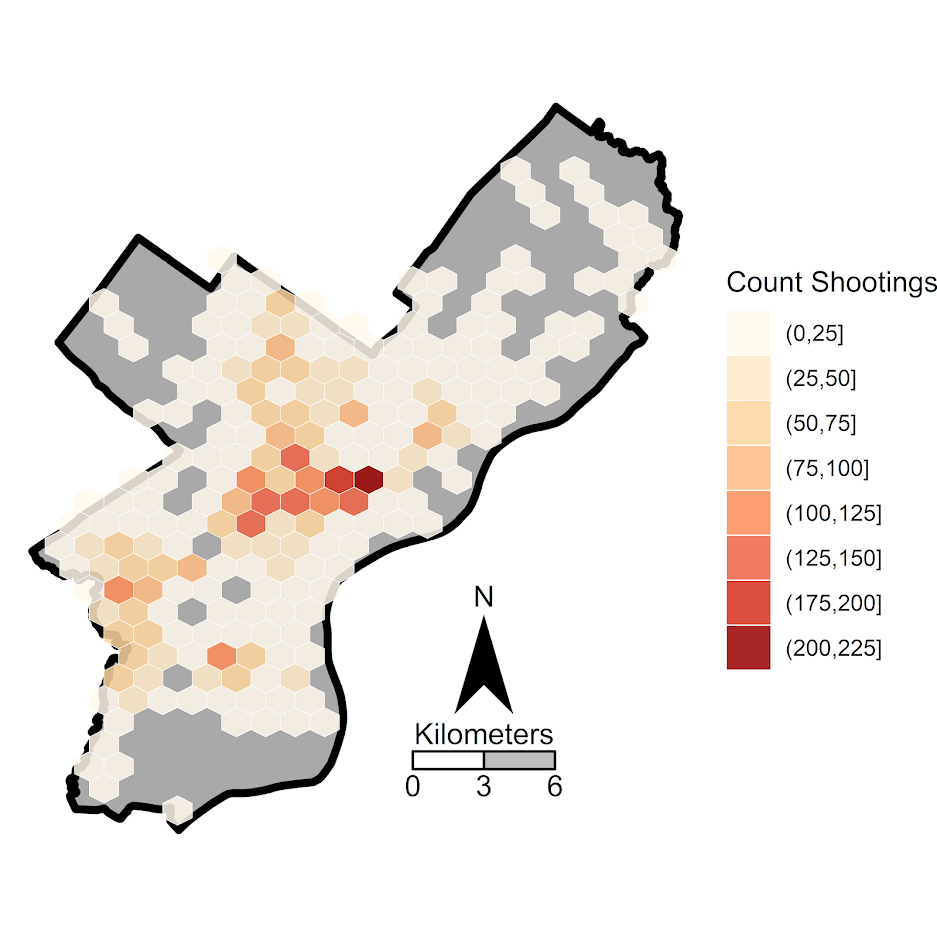# Making nice margin plots in Stata

For a recent working paper I had a student of mine (Jordan Riddell) help write some code to make nice margin plots in Stata, based on the work of Ben Jann and his grstyle code. Another good resource is Trenton Mize’s Sociological Science article on non-linear interactions. Here is what the end output will look like.My notes on how to get this to follow. Data and code to follow along can be downloaded from here.

## Start Up

First in my do file, I have a typical start up that sets the working directory and logs the results to a text file. I use `set more off` so I don’t have to do the annoying this and tell Stata to keep scrolling down. The next part is partly idiosyncratic to my Stata work set up — I call Stata from a centralized install location here at EPPS in UTD. I don’t have write access there, so to install commands I need to set my own place to install them on my local machine. So I add a location to `adopath` that is on my machine, and I also do `net set ado` to that same location.

Finally, for here I ssc installed `grstyle` and `palettes`. The code is currently commented out, as I only need to install it once. But it is good for others to know what extra packages they need to fully replicate your results.

``````**************************************************************************
*START UP STUFF

*Set the working directory and plain text log file
cd "C:\Users\axw161530\Dropbox\Documents\BLOG\Stata_NiceMargins\Analysis"

*log the results to a text file
log using "LogitModels.txt", text replace

*so the output just keeps going
set more off

*let stata know to search for a new location for stata plug ins

*In this script I use
*net install http://www.stata-journal.com/software/sj18-3/gr0073/
*ssc install grstyle, replace
*ssc install palettes, replace
**************************************************************************``````

## Graph Settings

Here is what I did to change my default graph settings. Again check out Ben Jann’s awesome website he made an all the great examples. That will be more productive than me commenting on every individual line.

``````**************************************************************************
*Graph Settings
grstyle clear
set scheme s2color
grstyle init
grstyle set plain, box
grstyle color background white
grstyle set color Set1
grstyle yesno draw_major_hgrid yes
grstyle yesno draw_major_ygrid yes
grstyle color major_grid gs8
grstyle linepattern major_grid dot
grstyle set legend 4, box inside
grstyle color ci_area gs12%50
**************************************************************************``````

## Data Prep

So here is pretty straight forward. I read in the data as a CSV file, generate a new variable that is the weekly average number of crimes within 1000 feet in the historical crime data (see the working paper for more details). One trick I like to use with regression models with many terms is to make a global that specifies those variables, so I don’t need to retype them a bunch. I named it `\$ContVars` here. Finally for simplicity in this script I am just examining the burglary incidents, so I get rid of the other crimes using the `keep` command.

``````**************************************************************************
*DATA PREP

*Getting the data
import delimited CrimeStrings_withData.csv

*Making the previous densities per time period
generate buff_1000_1 = buff_1000 * (7/1611)

*control variables used in the regression
global ContVars "d1 d2 d3 d4 d5 d6 d7 d8 d9 d10 d11 d12 d13 d14 d15 d16 d17 d18 whiteperc blackperc hispperc asianperc under17 propmove perpoverty perfemheadhouse perunemploy perassist i.month c.dateint"

*For here I am just examining burglary incidents
keep if crimetype == 3
**************************************************************************``````

## Making a Nice marginsplot

So basically what I want to do in the end is to draw an interaction effect between a dummy variable (whether a crime resulted in an arrest) and a continuous variable (the historical crime density at a location). I am predicting whether a crime results in a near-repeat follow up — hot spots with more crime on average will have more near-repeats simply by chance.

When displaying that interaction effect though, I only want to limit it to the support of the historical crime density in the sample. Or stated another way, the historical crime density variable basically ranges from 0 to 2.5 in the sample — I don’t care what the interaction effect is then at a historical crime density of 3.

To do that in Stata, I use `summarize` to get the min/max of that historical crime density and pipe them into a global. The Grid global will then tell Stata how often to calculate those effects. Too few and the plot may not look smooth, too many and it will take margins forever to calculate the results. Here 100 points is plenty.

``````*I will need this later to draw the margins over the support
*Of the prior crime density
summarize buff_1000_1
global MyMin = r(min)
global MyMax = r(max)
global Grid = (\$MyMax-\$MyMin)/100``````

This may seem overkill, as I could just fill in those values by hand later. If you look at my replication code for my paper though, I ended up doing this same thing for four different crimes and two different estimates, so I wanted as automated approach that avoids as many magic numbers as possible.

Now I estimate my logistic regression model, with my interaction effect and the global `\$ContVars` control variables I specified earlier. Here I am predicting whether a burglary has a follow up near-repeat crime (within 1000 feet and 7 days). I think an arrest will reduce that probability.

``````*Now estimate the logit model
logit future0_1000_7 i.arrest c.buff_1000_1 i.arrest#c.buff_1000_1 \$ContVars``````

Note that the estimate of the interaction effect looks like this:

``````--------------------------------------------------------------------------------------
future0_1000_7 |      Coef.   Std. Err.      z    P>|z|     [95% Conf. Interval]
---------------------+----------------------------------------------------------------
1.arrest |  -.0327821    .123502    -0.27   0.791    -.2748415    .2092774
buff_1000_1 |   1.457795   .0588038    24.79   0.000     1.342542    1.573048
|
arrest#c.buff_1000_1 |
1  |  -.5013652   .2742103    -1.83   0.067    -1.038807    .0360771
``````

So how exactly do I interpret this? It is very difficult to interpret the coefficients directly — it is much easier to make graphs and visualize what those effects actually mean on the probability of a near-repeat burglary occurring.

Now the good stuff. Basically I want to show the predicted probability of a near-repeat follow up crime, conditional on whether an arrest occurred, as well as the historical crime density. The first line uses `quietly`, so I don’t get the full margins table in the output. The second is just all the goodies to make my nice grey scale plot. Note I name the plot — this will be needed for later combining multiple plots.

``````*Create the two margin plots
quietly margins arrest, at(c.buff_1000_1=(\$MyMin (\$Grid) \$MyMax))
marginsplot, recast(line) noci title("Residential Burglary, Predictive Margins") xtitle("Historical Crime Density") ytitle("Pr(Future Crime = 1)") plot1opts(lcolor(black)) plot2opts(lcolor(gs6) lpattern("--")) legend(on order(1 "no arrest" 2 "arrest")) name(main)``````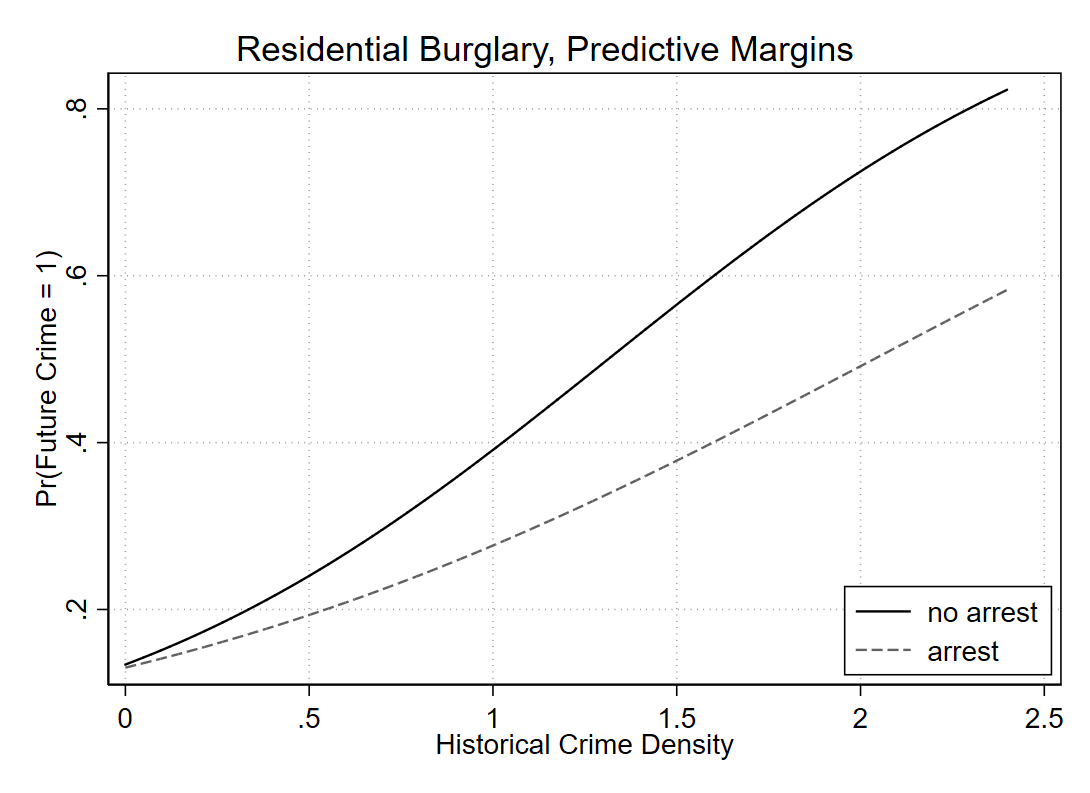You could superimpose confidence intervals on the prior plot, but those are the pointwise intervals for the probability for each individual line, they don’t directly tell you about the difference between the two lines. Viz. the difference in lines often can lead to misinterpretation (e.g. remember the Cleveland example of viz. the differences in exports/imports originally drawn by Playfair). Also superimposing multiple error bands tend to get visually messy. So a solution is to directly graph the estimate of the difference between those two probabilities in a separate graph. (Another idea though I’ve seen is here, a CI of the difference set to the midpoint of the two lines.)

``````quietly margins, dydx(arrest) at(c.buff_1000_1=(\$MyMin (\$Grid) \$MyMax))
marginsplot, recast(line) plot1opts(lcolor(gs8)) ciopt(color(black%20)) recastci(rarea) title("Residential Burglary, Average Marginal Effects of Arrest") xtitle("Historical Crime Density") ytitle("Effects on Pr(Future Crime)") name(diff)``````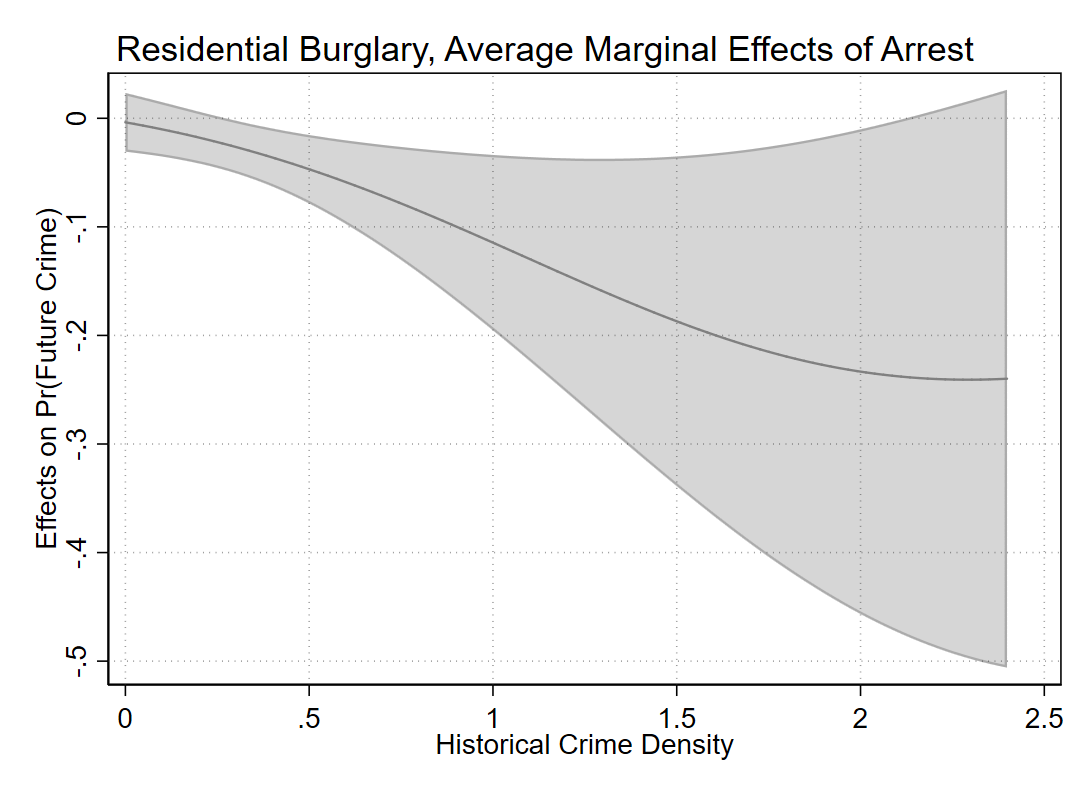Yay for the fact that Stata can now draw transparent areas. So here we can see that even though the marginal effect grows at higher prior crime densities — suggesting an arrest has a larger effect on reducing near repeats in hot spots, the confidence interval of the difference grows larger as well.

To end I combine the two plots together (same image at the beginning of the post), and then export them to a higher resolution PNG.

``````*Now combining the plots together
graph combine main diff, xsize(6.5) ysize(2.7) iscale(.8) name(comb)
graph close main diff
graph export "BurglaryMarginPlot.png", width(6000) replace``````I am often doing things interactively in the Stata shell when I am writing up scripts. Including redoing charts. To be able to redo a chart with the same name, you need to not only use `graph close`, but also `graph drop` it from memory. Then just dropping all the data and using `exit` will finish out your script and close down Stata entirely.

``````**************************************************************************
*FINISHING UP THE SCRIPT

*closing the combined graph
graph close comb

*This is necessary if you want to reuse the plot names
graph drop _all

*Finish the script.
drop _all
exit, clear
**************************************************************************  ``````

# Plotting Predictive Crime Curves

Writing some notes on this has been in the bucket list for a bit, how to evaluate crime prediction models. A recent paper on knife homicides in London is a good use case scenario for motivation. In short, when you have continuous model predictions, there are a few different graphs I would typically like to see, in place of accuracy tables.

The linked paper does not provide data, so what I do for a similar illustration is grab the lower super output area crime stats from here, and use the 08-17 data to predict homicides in 18-Feb19. I’ve posted the SPSS code I used to do the data munging and graphs here — all the stats could be done in Excel though as well (just involves sorting, cumulative sums, and division). Note this is not quite a replication of the paper, as it includes all cases in the homicide/murder minor crime category, and not just knife crime. There ends up being a total of 147 homicides/murders from 2018 through Feb-2019, so the nature of the task is very similar though, predicting a pretty rare outcome among almost 5,000 lower super output areas (4,831 to be exact).

So the first plot I like to make goes like this. Use whatever metric you want based on historical data to rank your areas. So here I used assaults from 08-17. Sort the dataset in descending order based on your prediction. And then calculate the cumulative number of homicides. Then calculate two more columns; the total proportion of homicides your ranking captures given the total proportion of areas.

Easier to show than to say. So for reference your data might look something like below (pretend we have 100 homicides and 1000 areas for a simpler looking table):

`````` PriorAssault  CurrHom CumHom PropHom PropArea
1000          1         1      1/100    1/1000
987          0         1      1/100    2/1000
962          2         4      4/100    3/1000
920          1         5      5/100    4/1000
.          .         .       .        .
.          .         .       .        .
.          .         .       .        .
0          0       100    100/100 1000/1000``````

You would sort the `PriorCrime` column, and then calculate `CumHom` (Cumulative Homicides), `PropHom` (Proportion of All Homicides) and `PropArea` (Proportion of All Areas). Then you just plot the `PropArea` on the X axis, and the `PropHom` on the Y axis. Here is that plot using the London data.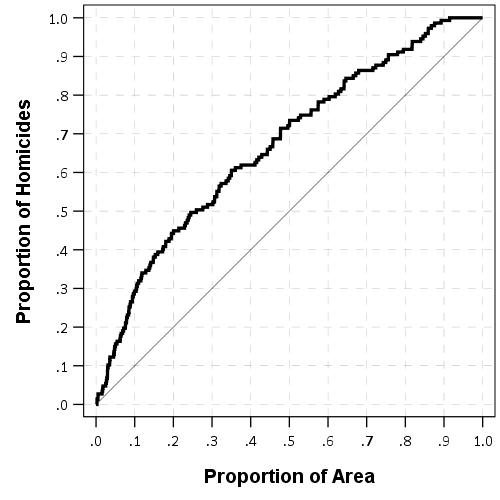Paul Ekblom suggests plotting the ROC curve, and I am too lazy now to show it, but it is very similar to the above graph. Basically you can do a weighted ROC curve (so predicting areas with more than 1 homicide get more weight in the graph). (See Mohler and Porter, 2018 for an academic reference to this point.)

Here is the weighted ROC curve that SPSS spits out, I’ve also superimposed the predictions generated via prior homicides. You can see that prior homicides as the predictor is very near the line of equality, suggesting prior homicides are no better than a coin-flip, whereas using all prior assaults does alittle better job, although not great. SPSS gives the area-under-the-curve stat at 0.66 with a standard error of 0.02.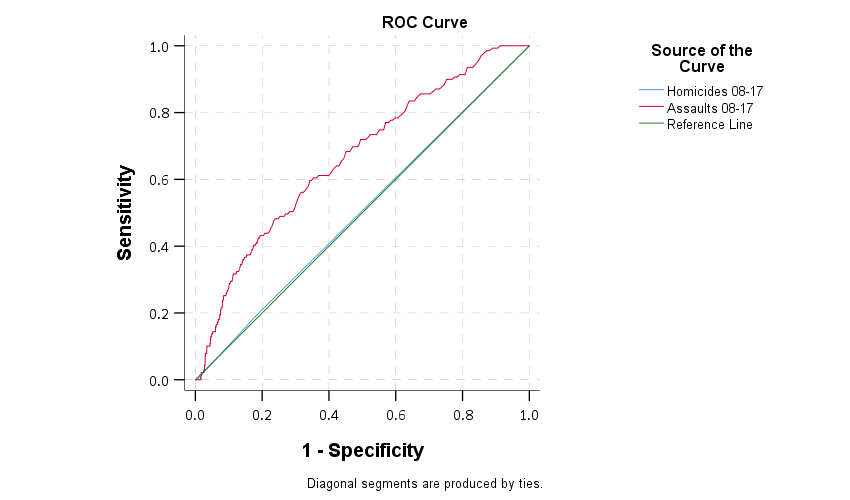Note that the prediction can be anything, it does not have to be prior crimes. It could be predictions from a regression model (like RTM), see this paper of mine for an example.

So while these do an OK job of showing the overall predictive ability of whatever metric — here they show using assaults are better than random, it isn’t real great evidence that hot spots are the go to strategy. Hot spots policing relies on very targeted enforcement of a small number of areas. The ROC curve shows the entire area. If you need to patrol 1,000 LSOA’s to effectively capture enough crimes to make it worth your while I wouldn’t call that hot spots policing anymore, it is too large.

So another graph you can do is to just plot the cumulative number of crimes you capture versus the total number of areas. Note this is based on the same information as before (using rankings based on assaults), just we are plotting whole numbers instead of proportions. But it drives home the point abit better that you need to go to quite a large number of areas to be able to capture a substantive number of homicides. Here I zoom in the plot to only show the first 800 areas.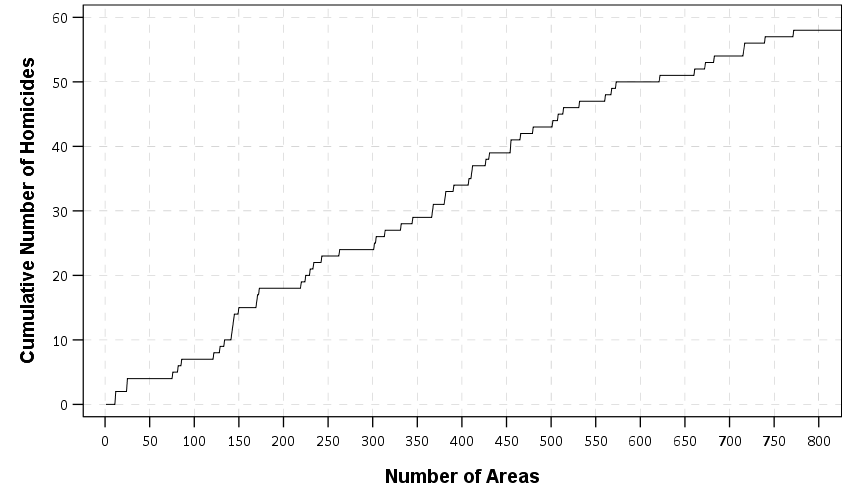So even though the overall curve shows better than random predictive ability, it is unclear to me if a rare homicide event is effectively concentrated enough to justify hot spots policing. Better than random predictions are not necessarily good enough.

A final metric worth making note of is the Predictive Accuracy Index (PAI). The PAI is often used in evaluating forecast accuracy, see some of the work of Spencer Chainey or Grant Drawve for some examples. The PAI is simply `% Crime Captured/% Area`, which we have already calculated in our prior graphs. So you want a value much higher than 1.

While those cited examples again use tables with simple cut-offs, you can make a graph like this to show the PAI metric under different numbers of areas, same as the above plots.The saw-tooth ends up looking very much like a precision-recall curve, but I haven’t sat down and figured out the equivalence between the two as of yet. It is pretty noisy, but we might have two regimes based on this — target around 30 areas for a PAI of 3-5, or target 150 areas for a PAI of 3. PAI values that low are not something to brag to your grandma about though.

There are other stats like the predictive efficiency index (PAI vs the best possible PAI) and the recapture-rate index that you could do the same types of plots with. But I don’t want to put everyone to sleep.

# Sorting rates using empirical Bayes

A problem I have come across in a few different contexts is the idea of ranking rates. For example, say a police department was interested in increasing contraband recovery and are considering two different streets to conduct additional traffic enforcement on — A and B. Say street A has a current hit rate of 50/1000 for a rate of 5%, and street B has a recovery rate of 1/10 for 10%. If you just ranked by percentages, you would choose street B. But given the small sample size, targeting street B is not a great bet to actually have a 10% hit rate going forward, so it may be better to choose street A.

The idea behind this observation is called shrinkage. Your best guess for the hit rate in either location A or location B in the future is not the observed percentage, but somewhere in between the observed percentage and the overall hit rate. Say the overall hit rate for contraband recovery is only 1%, then you wouldn’t expect street B to have a 10% hit rate going forward, but maybe something closer to 2% given the very small sample size. For street A you would expect shrinkage as well, but given it is a much larger sample size you would expect the shrinkage to be much less, say a 4% hit rate going forward. In what follows I will show how to calculate that shrinking using a technique called empirical Bayesian estimation.

I wanted to apply this problem to a recent ranking of cities based on officer involved shooting rates via federalcharges.com (hat tip to Justin Nix for tweeting that article). The general idea is that you don’t want to highlight cities who have high rates simply by chance due to smaller population baselines. Howard Wainer talks about this problem of ranking resulted in the false idea that smaller schools were better based on small samples of test results. Due to the high variance small schools will be both at the top and the bottom of the distributions, even if all of the schools have the same overall mean rate. Any reasonable ranking needs to take that variance into account to avoid the same mistake.

The same idea can be applied to homicide or other crime rates. Here I provide some simple code (and a spreadsheet) so other analysts can easily replicate this sorting idea for their own problems.

# Sorting OIS Shooting Rates

For this analysis I just took the reported rates by the federal changes post already aggregated to city, and added in 2010 census estimates from Wikipedia. I’d note these are not necessarily the correct denominator, some jurisdictions may cover less/more of the pop that these census designated areas. (Also you may consider other non-population denominators as well.) But just as a proof of concept I use the city population (which I suspect is what the original federal charges blog post used.)

The below graph shows the city population on the X axis, and the OIS rate per 100,000 on the Y axis. I also added in the average rate within these cities (properly taking into account that cities are different population sizes), and curves to show the 99% confidence interval funnel. You can see that the distribution is dispersed more than would be expected by the simple binomial proportions around the overall rate of close to 9 per 100,000.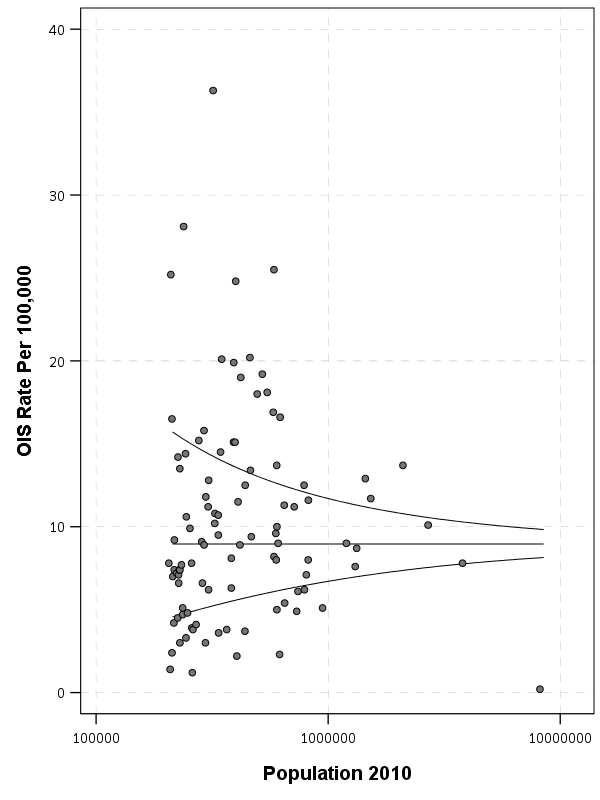The following section I have some more notes on how I calculated the shrinkage, but here is a plot that shows the original rate, and the empirical Bayes shrunk OIS rate. The arrow points to the shrunk rate, so you can see that places with smaller population proportions and those farther away from the overall rate are shrunk towards the overall OIS rate within this sample.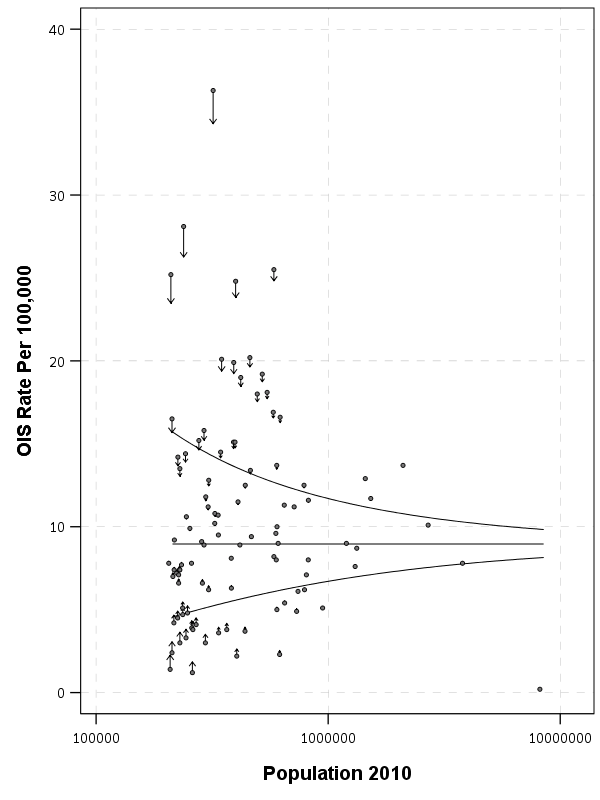To see how this changes the rankings, here is a slopegraph of the before/after rankings.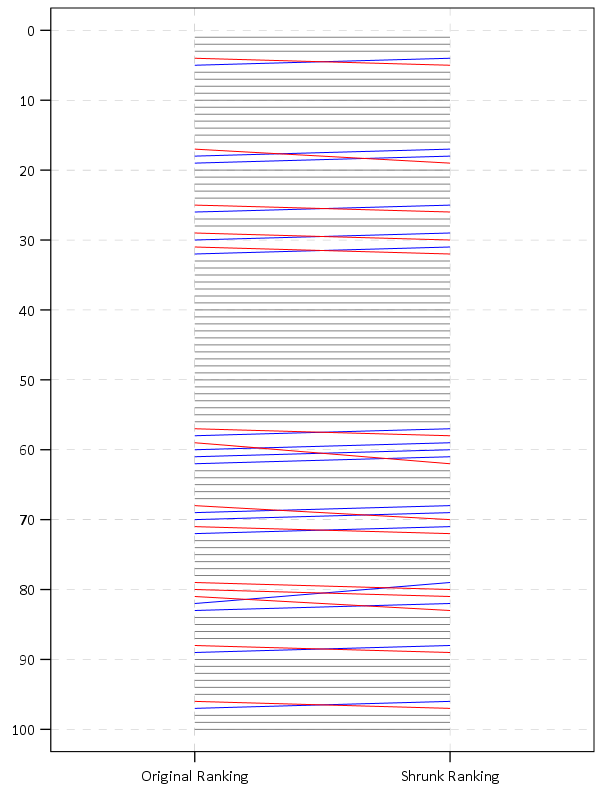So most of the rankings only change slightly using this technique. But if one incorporated cities with smaller populations though they would change even more.

The federal charges post also calculates differences in the OIS rate versus the homicide rate. That approach suffers from even worse problems in ignoring the variance of smaller population denominators (it compounds two high variance estimates), but I think the idea of adjusting for homicide rates in this context maybe has potential in a random effects binomial model (either as a covariate or a multivariate outcome). Would need to think about it/explore it some more though. Also to note is that the fatal encounters data is multiple years, so don’t be confused that OIS rates by police are larger than yearly homicide rates.

# The Mathy Part, Empirical Bayes Shrinkage

There are a few different ways I have seen reported to do empirical Bayes shrinkage. One is estimating the beta distribution for the entire sample, and then creating a shrunk estimate for the observed rates for individual observations using the observed sample Beta estimates as a prior (hence empirical Bayes). David Robinson has a nice little e-book on batting averages and empirical Bayes that can be applied to basically any type of percentage estimate you are interested in.

Another way I have seen it expressed is based on the work of the Luc Anselin and the GeoDa folks using explicit formulas.

Either of these ways you can do in a spreadsheet (a more complicated way is to actually fit a random effects model), but here is a simpler run-down of the GeoDa formula for empirical shrinkage, which is what I use in the above example. (This will not necessarily be the same compared to David Robinson’s approach, see the R code in the zip file of results for comparisons to David’s batting average dataset, but are pretty similar for that example.) So you can think of the shrunk rate as a weighted average between the observed rate for location `i` as `y_i`, and the overall rate `mu`, where the weight is `W_i`.

``Shrunk Rate_i = W_i*y_i + (1 - W_i)*mu``

You then need to calculate the `W_i` weight term. Weights closer to 1 (which will happen with bigger population denominators) result in only alittle shrinkage. Weights closer to 0 (when the population denominator is small), result in much larger shrinkage. Below are the formulas and variable definitions to calculate the shrinkage.

• `i = subscript to denote area i. No subscript means it is a scalar.`
• `r_i = total number of incidents (numerator) in area i`
• `p_i = total population in area i (denominator)`
• `y_i = observed rate in area i = r_i/p_i`
• `k = total number of areas in study`
• `mu = population mean rate = sum(r_i)/sum(p_i)`
• `v = population variance = sum(p_i*[y_i - mu]^2]) / [sum(p_i)] - mu/(sum(p_i)/k)`
• `W_i = shrinkage weight = v /[v + (mu/p_i)]`

For those using R, here is a formula that takes the numerator and denominator as vectors and returns the smoothed rate based on the above formula:

``````#R function
shrunkrate <- function(num,den){
sDen <- sum(den)
obsrate <- num/den
k <- length(num)
mu <- sum(num)/sDen
pav <- sDen/k
v <- ( sum( den*(obsrate-mu)^2 ) / sDen ) - (mu/pav)
W <- v / (v + (mu/den))
smoothedrate <- W*obsrate + (1 - W)*mu
return(smoothedrate)
}``````

For those using SPSS I’ve uploaded macro code to do both the funnel chart lines and the shrunk rates.

For either missing values might mess things up, so eliminate them before using the functions. For those who don’t use stat software, I have also included an Excel spreadsheet that shows how to calculate the smoothed rates. It is in this zip file, along with other code and data used to replicate my graphs and results here.

For those interested in other related ideas, see

# Making interactive plots with R and Plotly

I wrote a small op-ed based on the homicide studies work I recently published about interpreting crime trends. Unfortunately that op-ed was not picked up by anyone (I missed the timing abit, maybe next year when the UCR stats come out I can just update the numbers and make the same point). I’ve posted that op-ed here, and I wanted to make a quick blog post detailing how I made the interactive graphs in that post using R and the Plotly library. All the data and code to replicate this can be downloaded from here.

Unfortunately with my free wordpress blog I cannot embed the actual interactive graphics, but I will provide links to online versions at my UT Dallas page that work and show a screenshot of each. So first, lets load all of the libraries that you will need, as well as set the working directory. (Of course change it to where you have your data saved on your local machine.)

``````#########################################################
#Making a shiny app for homicide rate chart
library(shiny)
library(ggplot2)
library(plotly)
library(htmlwidgets)
library(scales)

mydir <- "C:\\Users\\axw161530\\Box Sync\\Projects\\HomicideGraphs\\Analysis\\Analysis"
setwd(mydir)
#########################################################``````

Now I just read in the data. I have two datasets, the funnel rates just has additional columns to draw the funnel graphs already created. (See here or here, or the data in the original Homicide Studies paper linked at the top, on how to construct these.)

``````############################################################
#Get the data

summary(FunnRates)
FunnRates\$Population <- FunnRates\$Pop1 #These are just to make nicer labels
FunnRates\$HomicideRate <- FunnRates\$HomRate

summary(IntRates)
############################################################``````

## Funnel Chart for One Year

First, plotly makes it dead easy to take graphs you created via ggplot and turn them into an interactive graph. So here is a link to the interactive chart, and below is a screenshot.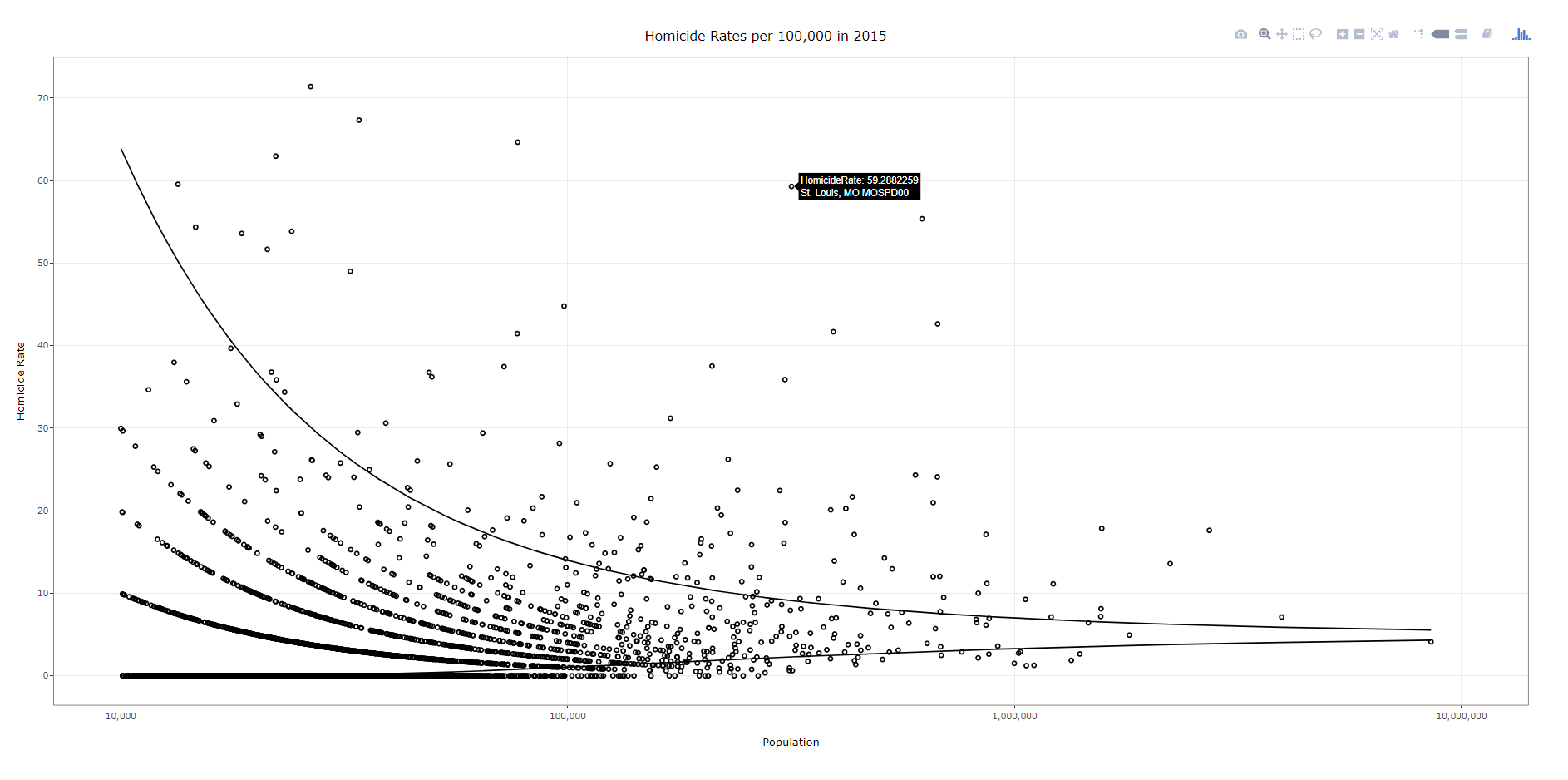To walk through the code, first you make your (almost) plane Jane ggplot object. Here I name it `p`. You will get an error for an “unknown aesthetics: text”, but this will be used by plotly to create tooltips. Then you use the `ggplotly` function to turn the original ggplot graph `p` into an interactive graph. By default the plotly object has more stuff in the tooltip than I want, which you can basically just go into the innards of the plotly object and strip out. Then the final part is just setting the margins to be alittle larger than default, as the axis labels were otherwise slightly cut-off.

``````############################################################
#Make the funnel chart
year_sel <- 2015
p <- ggplot(data = FunnRates[FunnRates\$Year == year_sel,]) + geom_point(aes(x=Population, y=HomicideRate, text=NiceLab), pch=21) +
geom_line(aes(x=Population,y=LowLoc99)) + geom_line(aes(x=Population,y=HighLoc99)) +
labs(title = paste0("Homicide Rates per 100,000 in ",year_sel)) +
scale_x_log10("Population", limits=c(10000,10000000), breaks=c(10^4,10^5,10^6,10^7), labels=comma) +
scale_y_continuous("Homicide Rate", breaks=seq(0,110,10)) +
theme_bw() #+ theme(text = element_text(size=20), axis.title.y=element_text(margin=margin(0,10,0,0)))

pl <- ggplotly(p, tooltip = c("HomicideRate","text"))
#pl <- plotly_build(p, width=1000, height=900)
#See https://stackoverflow.com/questions/45801389/disable-hover-information-for-a-specific-layer-geom-of-plotly
pl\$x\$data[]\$hoverinfo <- "none"
pl\$x\$data[]\$hoverinfo <- "none"
pl <- pl %>% layout(margin = list(l = 75, b = 65))
############################################################``````

After this point you can just type `pl` into the console and it will open up an interactive window. Or you can use the `saveWidget` function from the htmlwidgets package, something like `saveWidget(as_widget(pl), "FunnelChart_2015.html", selfcontained=TRUE)` to save the graph to an html file.

Now there are a couple of things. You can edit various parts of the graph, such as its size and label text size, but depending on your application these might not be a good idea. If you need to take into account smaller screens, I think it is best to use some of the defaults, as they adjust per the screen that is in use. For the size of the graph if you are embedding it in a webpage using iframe’s you can set the size at that point. If you look at my linked op-ed you can see I make the funnel chart taller than wider — that is through the iframe specs.

## Funnel Chart over Time

Ok, now onto the fun stuff. So we have a funnel chart for one year, but I have homicide years from 1965 through 2015. Can we examine those over time. Plotly has an easy to use additional argument to ggplot graphs, named `Frame`, that allows you to add a slider to the interactive chart for animation. The additional argument `ids` links one object over time, ala Hans Rosling bubble chart over time. Here is a link to the interactive version, and below is a screen shot: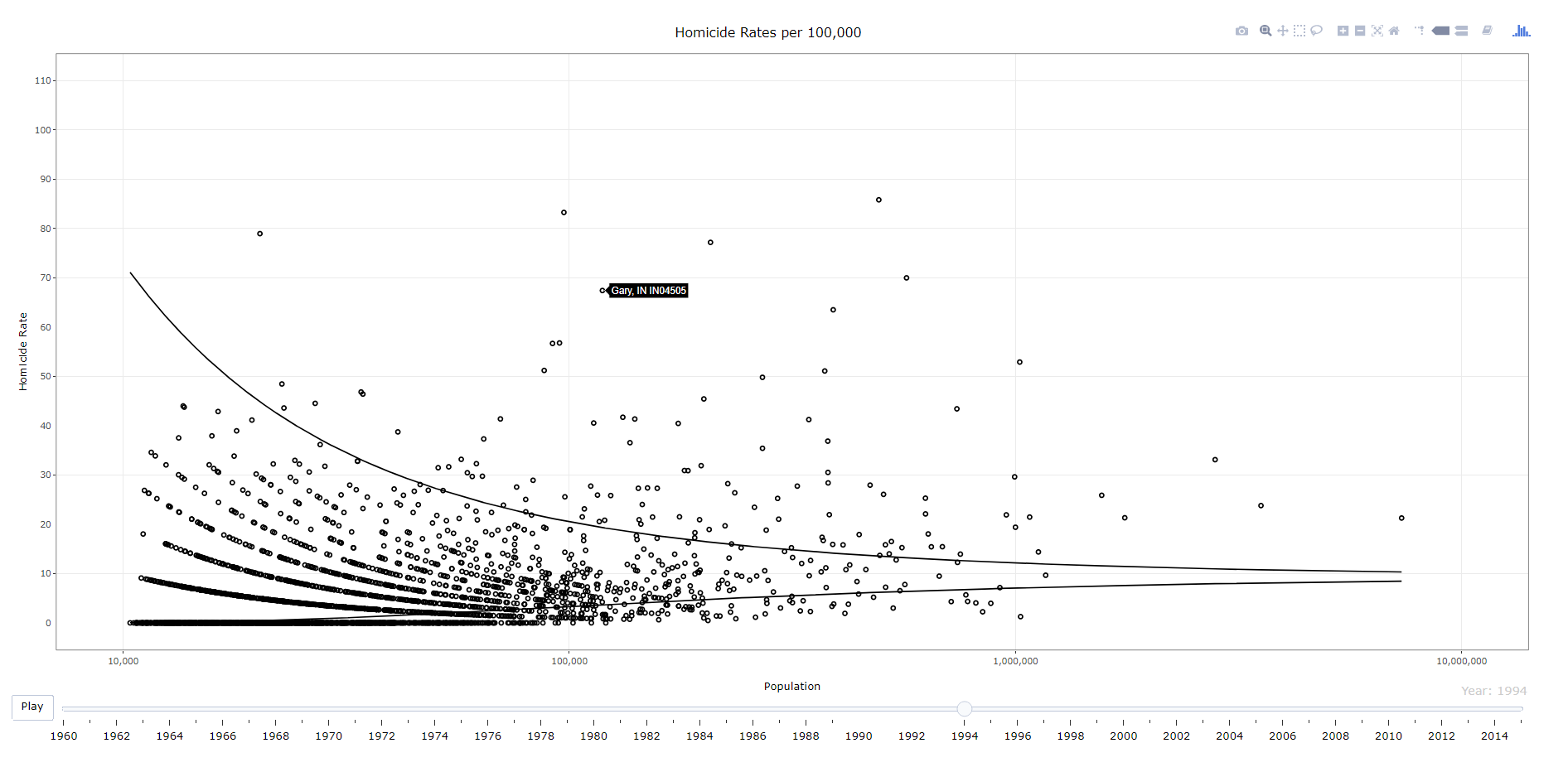``````############################################################
#Making the funnel chart where you can select the year
py <- ggplot(data = FunnRates) + geom_point(aes(x=Population, y=HomicideRate, text=NiceLab, frame=Year,ids=ORI), pch=21) +
geom_line(aes(x=Population,y=LowLoc99,frame=Year)) + geom_line(aes(x=Population,y=HighLoc99,frame=Year)) +
labs(title = paste0("Homicide Rates per 100,000")) +
scale_x_log10("Population", limits=c(10000,10000000), breaks=c(10^4,10^5,10^6,10^7), labels=comma) +
scale_y_continuous("Homicide Rate", breaks=seq(0,110,10), limits=c(0,110)) +
theme_bw() #+ theme(text = element_text(size=20), axis.title.y=element_text(margin=margin(0,10,0,0)))

ply <- ggplotly(py, tooltip = c("text")) %>% animation_opts(0, redraw=FALSE)
ply\$x\$data[]\$hoverinfo <- "none"
ply\$x\$data[]\$hoverinfo <- "none"
saveWidget(as_widget(ply), "FunnelChart_YearSelection.html", selfcontained=FALSE)
############################################################``````

The way I created the data it does not make sense to do a smooth animation for the funnel line, so this just flashes to each new year (via the `animation_opts` spec). (I could make the data so it would look nicer in an animation, but will wait for someone to pick up the op-ed before I bother too much more with this.) But it accomplishes via the slider the ability for you to pick which year you want.

## Fan Chart Just One City

Next we are onto the fan charts for each individual city with the prediction intervals. Again you can just create this simple chart in ggplot, and then use plotly to make a version with tooltips. Here is a link to an interactive version, and below is a screenshot.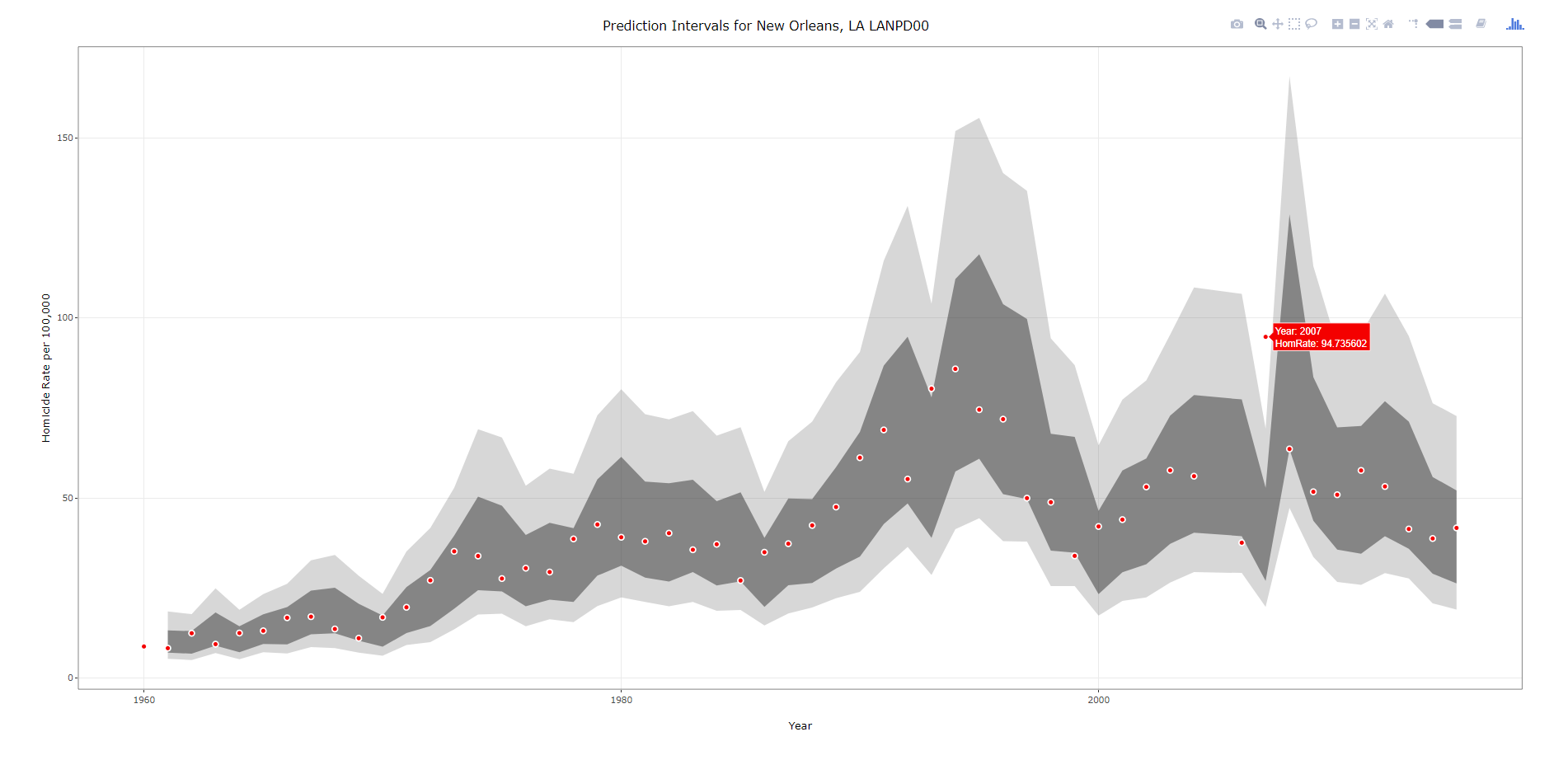``````###################################################
#Making the fan graph for New Orleans
titleLab <- unique(IntRates[,c("ORI","NiceLab","AgencyName","State")])
p2 <- ggplot(data=IntRates[IntRates\$ORI == "LANPD00",], aes(x=Year, y=HomRate)) +
geom_ribbon(aes(ymin=LowB, ymax=HighB), alpha=0.2) +
geom_ribbon(aes(ymin=LagLow25, ymax=LagHigh25), alpha=0.5) +
geom_point(shape=21, color="white", fill="red", size=2) +
labs(x = "Year", y="Homicide Rate per 100,000") +
#scale_x_continuous(breaks=seq(1960,2015,by=5)) +
ggtitle(paste0("Prediction Intervals for ",titleLab[titleLab\$ORI == "LANPD00",c("NiceLab")])) +
theme_bw() #+ theme(text = element_text(size=20), axis.title.y=element_text(margin=margin(0,10,0,0)))
#p2
pl2 <- ggplotly(p2, tooltip = c("Year","HomRate"), dynamicTicks=TRUE)
pl2\$x\$data[]\$hoverinfo <- "none"
pl2\$x\$data[]\$hoverinfo <- "none"
pl2 <- pl2 %>% layout(margin = list(l = 100, b = 65))
#pl2
saveWidget(as_widget(pl2), "FanChart_NewOrleans.html", selfcontained=FALSE)
###################################################``````

Note when you save the widget to `selfcontained=FALSE`, it hosts several parts of the data into separate folders. I always presumed this was more efficient than making one huge html file, but I don’t know for sure.

## Fan Chart with Dropdown Selection

Unfortunately the `frame` type animation does not make as much sense here. It would be hard for someone to find a particular city of interest in that slider (as a note though the slider can have nominal data, if I only had a few cities it would work out ok, with a few hundred it will not though). So feature request if anyone from plotly is listening — please have a dropdown type option for ggplot graphs! In the meantime though there is an alternative using a tradition `plot_ly` type chart. Here is that interactive fan chart with a police agency dropdown, and below is a screenshot.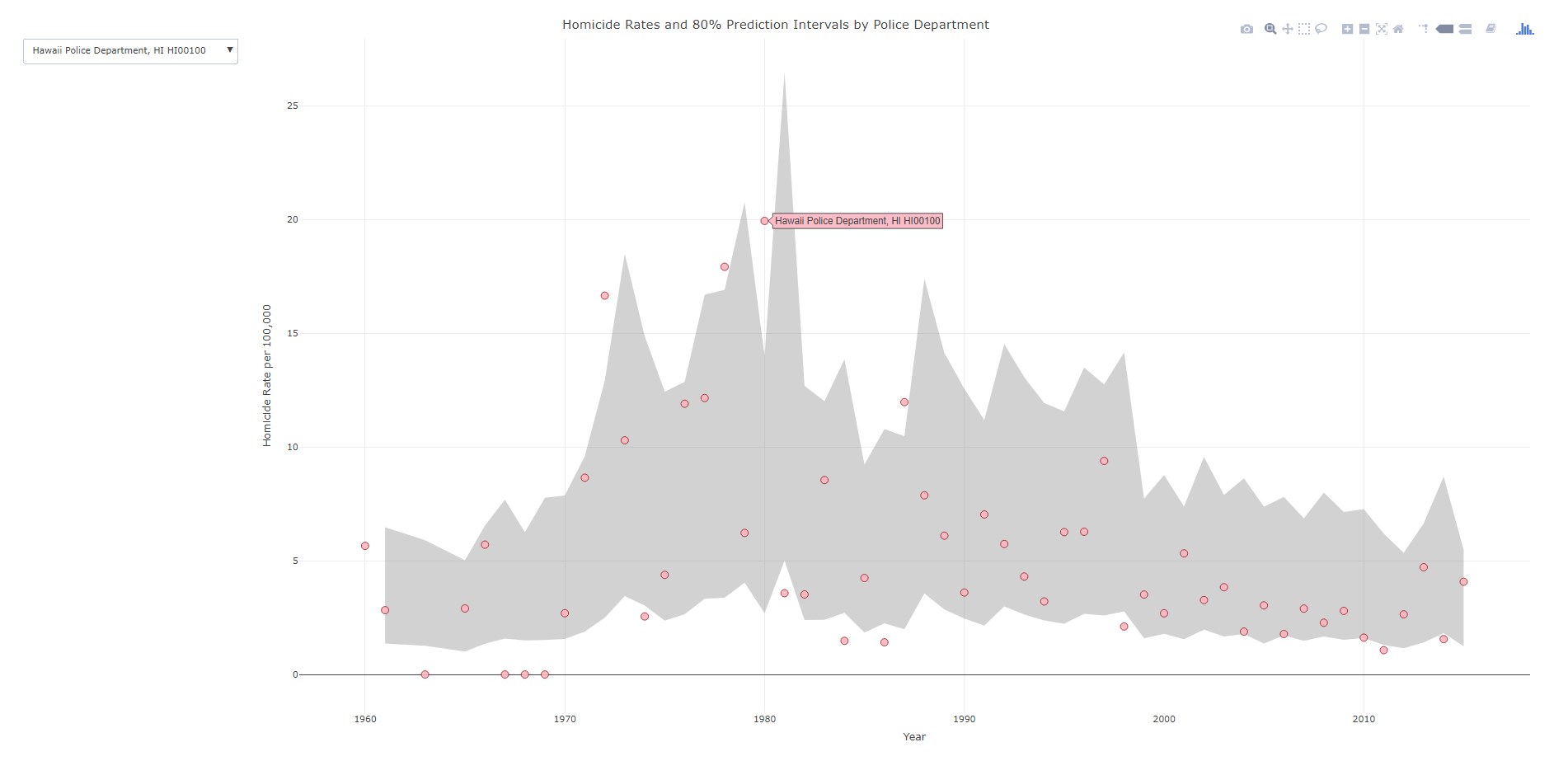``````###################################################
#Making the fan graph where you can select the city of interest
#Need to have a dropdown for the city

titleLab <- unique(IntRates[,c("ORI","NiceLab","State")])
nORI <- length(titleLab[,1])
choiceP <- vector("list",nORI)
for (i in 1:nORI){
choiceP[[i]] <- list(method="restyle", args=list("transforms.value", unique(IntRates\$NiceLab)[i]), label=titleLab[i,c("NiceLab")])
}

trans <- list(list(type='filter',target=~NiceLab, operation="=", value=unique(IntRates\$NiceLab)))
textLab <- ~paste("Homicide Rate:",HomRate,'\$
Year:',Year,'\$
Homicides:',Homicide,'\$
Population:',Pop1,'\$
Agency Name:',NiceLab)

#Lets try with the default plotly
#See https://community.plot.ly/t/need-help-on-using-dropdown-to-filter/6596
ply4 <- IntRates %>%
plot_ly(x= ~Year,y= ~HighB, type='scatter', mode='lines', line=list(color='transparent'), showlegend=FALSE, name="90%", hoverinfo="none", transforms=trans) %>%
add_trace(y=~LowB,  type='scatter', mode='lines', line=list(color='transparent'), showlegend=FALSE, name='10%', hoverinfo="none", transforms=trans,
fill = 'tonexty', fillcolor='rgba(105,105,105,0.3)') %>%
add_trace(x=~Year,y=~HomRate, text=~NiceLab, type='scatter', mode='markers', marker = list(size=10, color = 'rgba(255, 182, 193, .9)', line = list(color = 'rgba(152, 0, 0, .8)', width = 1)),
hoverinfo='text', text=textLab, transforms=trans) %>%
layout(title = "Homicide Rates and 80% Prediction Intervals by Police Department",
xaxis = list(title="Year"),
yaxis = list(title="Homicide Rate per 100,000"),

saveWidget(as_widget(ply4), "FanChart_Dropdown.html", selfcontained=FALSE)
###################################################``````

So in short plotly makes it super-easy to make interactive graphs with tooltips. Long term goal I would like to make a visual supplement to the traditional UCR report (I find the complaint of what tables to include to miss the point — there are much better ways to show the information that worrying about the specific tables). So if you would like to work on that with me always feel free to get in touch!

# New preprint: Testing for Similarity in Area-Based Spatial Patterns: Alternative Methods to Andresen’s Spatial Point Pattern Test

I just posted another pre-print to SSRN, Testing for Similarity in Area-Based Spatial Patterns: Alternative Methods to Andresen’s Spatial Point Pattern Test. This is work with Wouter Steenbeek and Martin Andresen. Below is the abstract:

Andresen’s spatial point pattern test (SPPT) compares two spatial point patterns on defined areal units: it identifies areas where the spatial point patterns diverge and aggregates these local (dis)similarities to one global measure. We discuss the limitations of the SPPT and provide two alternative methods to calculate differences in the point patterns. In the first approach we use differences in proportions tests corrected for multiple comparisons. We show how the size of differences matter, as with large point patterns many areas will be identified by SPPT as statistically different, even if those differences are substantively trivial. The second approach uses multinomial logistic regression, which can be extended to identify differences in proportions over continuous time. We demonstrate these methods on identifying areas where pedestrian stops by the New York City Police Department are different from violent crimes from 2006 through 2016.

And here is an example map using our proportion differences test and graduated circles to identify places with larger differences in the percentages:This is opposed to the traditional SPPT output, which just identifies whether two areas are different and does not focus on the size of the difference, like below: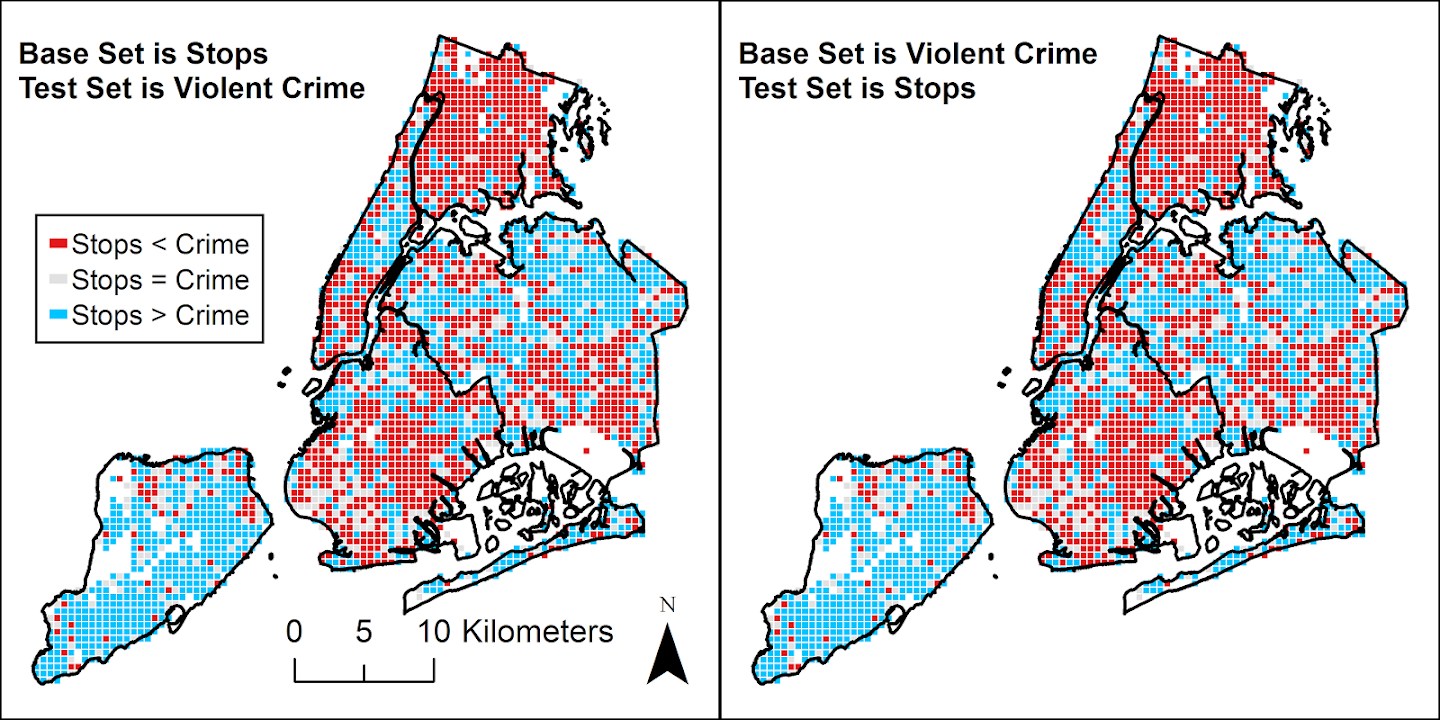You can see with a large sample size, basically everything is statistically different! (This uses over 4 million stops and over 800,000 violent crimes). Focusing on the magnitude of the differences gives a much clear indication of patterns.

The paper includes a dropbox link to download the data and code used to estimate the different techniques (it includes code in SPSS, R, and Stata). If you have any feedback as always let me know. This was submitted as a GISScience presentation for the 2018 ESRI User conference in July in San Diego, so I should have news about that presentation in the near future as well.

# Remaking a clustered bar chart

Thomas Lumley on his blog had a recent example of remaking a clustered bar chart that I thought was a good idea. Here is a screenshot of the clustered bar chart (the original is here):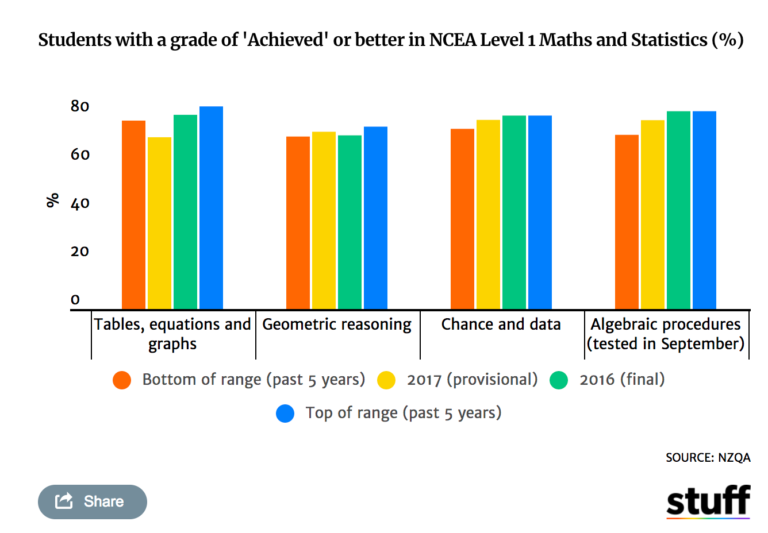And here is Lumley’s remake: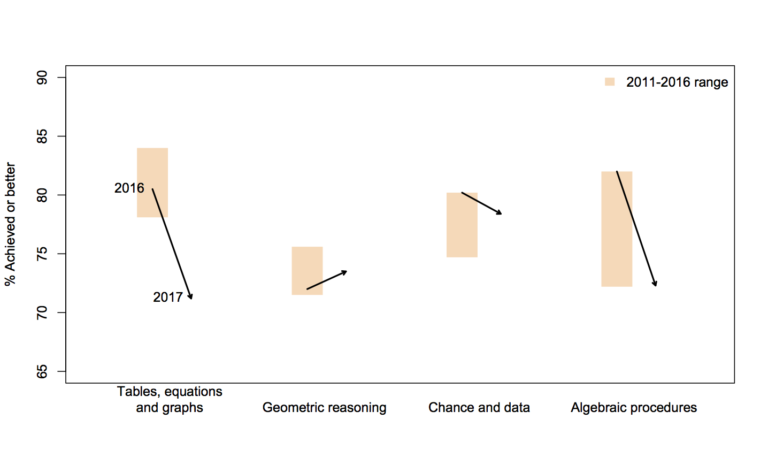In the original bar chart it is hard to know what is the current value (2017) and what are the past values. Also the bar chart goes to zero on the Y axis, which makes any changes seem quite small, since the values only range from 70% to 84%. Lumley’s remake clearly shows the change from 2016 to 2017, as well as the historical range from 2011 through 2016.

I like Lumley’s remake quite alot, so I made some code in SPSS syntax to show how to make a similar chart. The grammar of graphics I always thought is alittle confusing when using clustering, so this will be a good example demonstration. Instead of worrying about the legend I just added in text annotations to show what the particular elements were.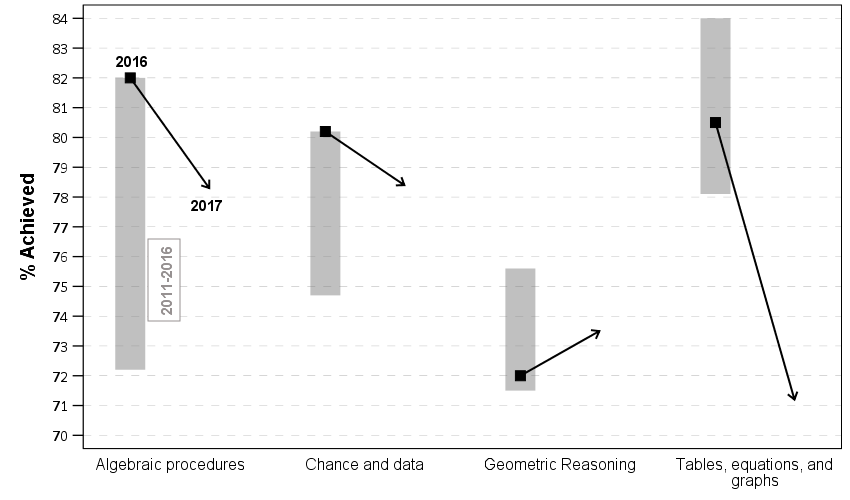One additional remake is instead of offsetting the points and using a slope chart (this is an ok use, but see my general critique of slopegraphs here) is to use a simpler dotplot showing before and after.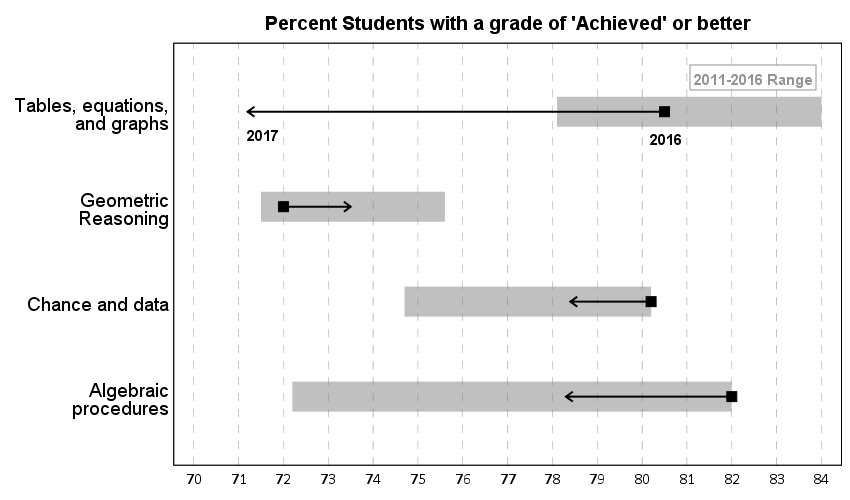One reason I do not like the slopes is that slope itself is dictated by the distance from 16 to 17 in the chart (which is arbitrary). If you squeeze them closer together the slope gets higher. The slope itself does not encode the data you want, you want to calculate the difference from beginning to end. But it is not a big difference here (my main complaints for slopegraphs are when you superimpose many different slopes that cross one another, in those cases I think a scatterplot is a better choice).

Jonathan Schwabish on his blog often has similar charts (see this one example).

Pretty much all clustered bar charts can be remade into either a dotplot or a line graph. I won’t go as far as saying you should always do this, but I think dot plots or line graphs would be a better choice than a clustered bar graph for most examples I have seen.

Here like Lumley said instead of showing the ranges likely a better chart would just be a line chart over time of the individual years, that would give a better since of both trends as well as typical year-to-year changes. But these alternatives to a clustered bar chart I do not think turned out too shabby.

SPSS Code to replicate the charts. I added in the labels for the elements manually.

``````**********************************************************************************************.
*data from https://www.stuff.co.nz/national/education/100638126/how-hard-was-that-ncea-level-1-maths-exam.
*Motivation from Thomas Lumley, see https://www.statschat.org.nz/2018/01/18/better-or-worse/.

DATA LIST FREE / Type (A10) Low Y2017 Y2016 High (4F3.1).
BEGIN DATA
Tables 78.1 71.2 80.5 84
Geo 71.5 73.5 72 75.6
Chance 74.7 78.4 80.2 80.2
Algebra 72.2 78.3 82 82
END DATA.
DATASET NAME Scores.
VALUE LABELS Type
'Tables' 'Tables, equations, and graphs'
'Geo' 'Geometric Reasoning'
'Chance' 'Chance and data'
'Algebra' 'Algebraic procedures'
.
FORMATS Low Y2017 Y2016 High (F3.0).
EXECUTE.

*In this format I can make a dot plot.
GGRAPH
/GRAPHDATASET NAME="graphdataset" VARIABLES=Y2017 Y2016 Low High Type
/GRAPHSPEC SOURCE=INLINE.
BEGIN GPL
SOURCE: s=userSource(id("graphdataset"))
DATA: Y2017=col(source(s), name("Y2017"))
DATA: Y2016=col(source(s), name("Y2016"))
DATA: Low=col(source(s), name("Low"))
DATA: High=col(source(s), name("High"))
DATA: Type=col(source(s), name("Type"), unit.category())
GUIDE: axis(dim(1), delta(1), start(70))
GUIDE: axis(dim(1), label("Percent Students with a grade of 'Achieved' or better"), opposite(), delta(100), start(60))
GUIDE: axis(dim(2))
SCALE: cat(dim(2), include("Algebra", "Chance", "Geo", "Tables"))
ELEMENT: edge(position((Low+High)*Type), size(size."30"), color.interior(color.grey),
transparency.interior(transparency."0.5"))
ELEMENT: edge(position((Y2016+Y2017)*Type), shape(shape.arrow), color(color.black), size(size."2"))
ELEMENT: point(position(Y2016*Type), color.interior(color.black), shape(shape.square), size(size."10"))
END GPL.

*Now trying a clustered bar graph.
GGRAPH
/GRAPHDATASET NAME="graphdataset" VARIABLES=Y2017 Y2016 Low High Type
/GRAPHSPEC SOURCE=INLINE.
BEGIN GPL
SOURCE: s=userSource(id("graphdataset"))
DATA: Y2017=col(source(s), name("Y2017"))
DATA: Y2016=col(source(s), name("Y2016"))
DATA: Low=col(source(s), name("Low"))
DATA: High=col(source(s), name("High"))
DATA: Type=col(source(s), name("Type"), unit.category())
TRANS: Y17 = eval("2017")
TRANS: Y16 = eval("2016")
COORD: rect(dim(1,2), cluster(3,0))
GUIDE: axis(dim(3))
GUIDE: axis(dim(2), label("% Achieved"), delta(1), start(70))
ELEMENT: edge(position(Y16*(Low+High)*Type), size(size."30"), color.interior(color.grey),
transparency.interior(transparency."0.5"))
ELEMENT: edge(position((Y16*Y2016*Type)+(Y17*Y2017*Type)), shape(shape.arrow), color(color.black), size(size."2"))
ELEMENT: point(position(Y16*Y2016*Type), color.interior(color.black), shape(shape.square), size(size."10"))
END GPL.

*This can get tedious if you need to make a line for many different years.
*Reshape to make a clustered chart in a less tedious way (but cannot use arrows this way).
VARSTOCASES /MAKE Perc FROM Y2016 Y2017 /INDEX Year.
COMPUTE Year = Year + 2015.
DO IF Year = 2017.
COMPUTE Low = \$SYSMIS.
COMPUTE High = \$SYSMIS.
END IF.
EXECUTE.

GGRAPH
/GRAPHDATASET NAME="graphdataset" VARIABLES=Type Perc Year Low High MISSING=VARIABLEWISE REPORTMISSING=NO
/GRAPHSPEC SOURCE=INLINE.
BEGIN GPL
SOURCE: s=userSource(id("graphdataset"))
DATA: Type=col(source(s), name("Type"), unit.category())
DATA: Perc=col(source(s), name("Perc"))
DATA: Low=col(source(s), name("Low"))
DATA: High=col(source(s), name("High"))
DATA: Year=col(source(s), name("Year"), unit.category())
COORD: rect(dim(1,2), cluster(3,0))
GUIDE: axis(dim(3))
GUIDE: axis(dim(2), label("% Achieved"), delta(1), start(70))
SCALE: cat(dim(3), include("Algebra", "Chance", "Geo", "Tables"))
ELEMENT: edge(position(Year*(Low+High)*Type), color.interior(color.grey), size(size."20"), transparency.interior(transparency."0.5"))
ELEMENT: path(position(Year*Perc*Type), split(Type))
ELEMENT: point(position(Year*Perc*Type), size(size."8"), color.interior(color.black), color.exterior(color.white))
END GPL.
**********************************************************************************************.``````

# New working paper: Mapping attitudes towards the police at micro places

I have a new preprint posted, Mapping attitudes towards the police at micro places. This is work with Jasmine Silver, as well as Rob Worden and Sarah McLean. See the abstract:

We demonstrate the utility of mapping community satisfaction with the police at micro places using data from citizen surveys conducted in 2001, 2009 and 2014 in one city. In each survey, respondents provided the nearest intersection to their address. We use inverse distance weighting to map a smooth surface of satisfaction with police over the entire city, which shows broader neighborhood patterns of satisfaction as well as small area hot spots of dissatisfaction. Our results show that hot spots of dissatisfaction with police do not conform to census tract boundaries, but rather align closely with hot spots of crime and police activity. Models predicting satisfaction with police show that local counts of violent crime are the strongest predictors of attitudes towards police, even above individual level predictors of race and age.

In this article we make what are analogs of hot spot maps of crime, but measure dissatisfaction with the police.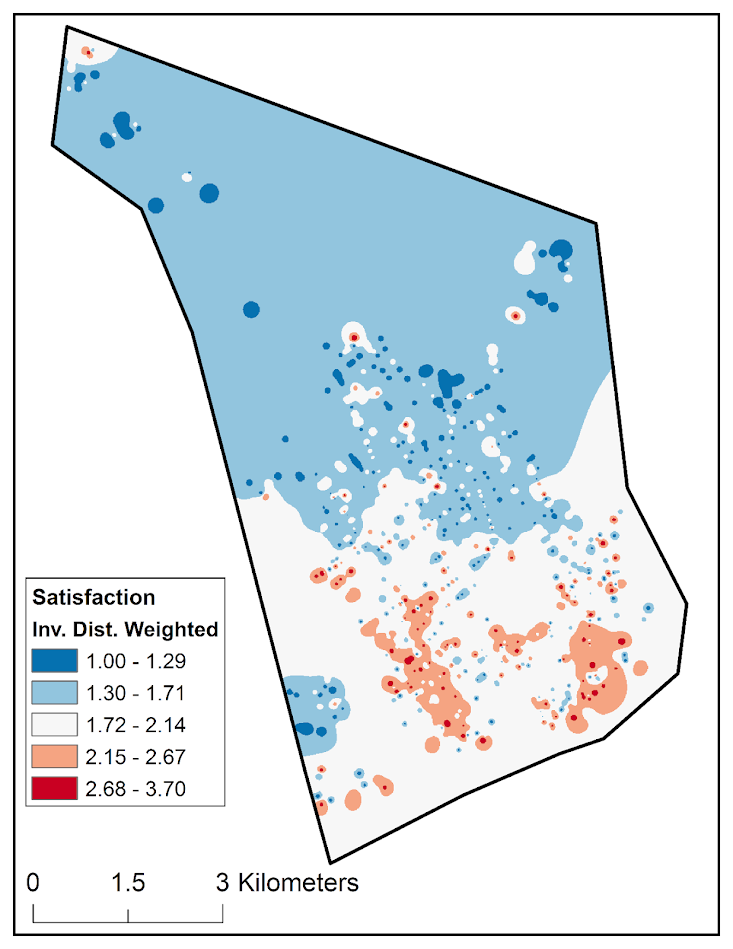One of the interesting findings is that these hot spots do not align nicely with census tracts (the tracts are generalized, we cannot divulge the location of the city). So the areas identified by each procedure would be much different.As always, feel free to comment or send me an email if you have feedback on the article.

# Monitoring homicide trends paper published

My paper, Monitoring Volatile Homicide Trends Across U.S. Cities (with coauthor Tom Kovandzic) has just been published online in Homicide Studies. Unfortunately, Homicide Studies does not give me a link to share a free PDF like other publishers, but you can either grab the pre-print on SSRN or always just email me for a copy of the paper.

They made me convert all of the charts to grey scale :(. Here is an example of the funnel chart for homicide rates in 2015.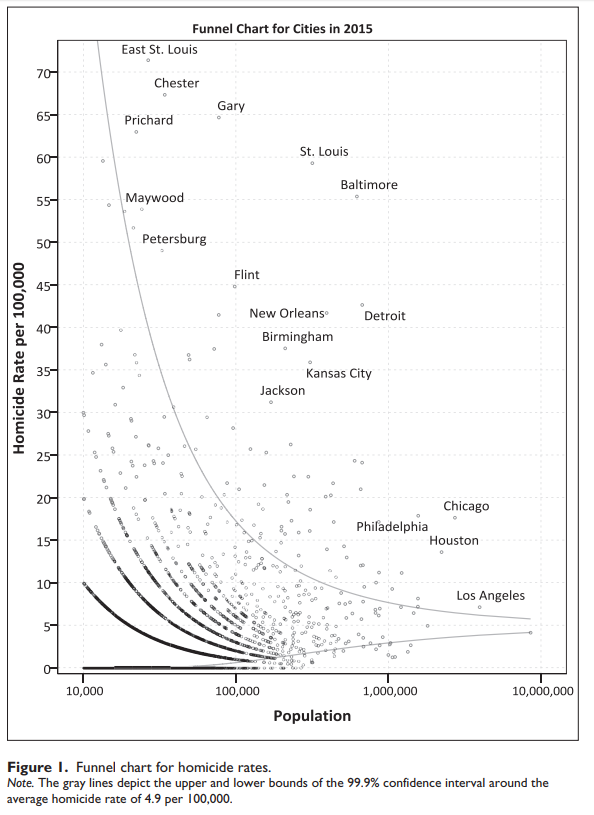And here are example fan charts I generated for a few different cities.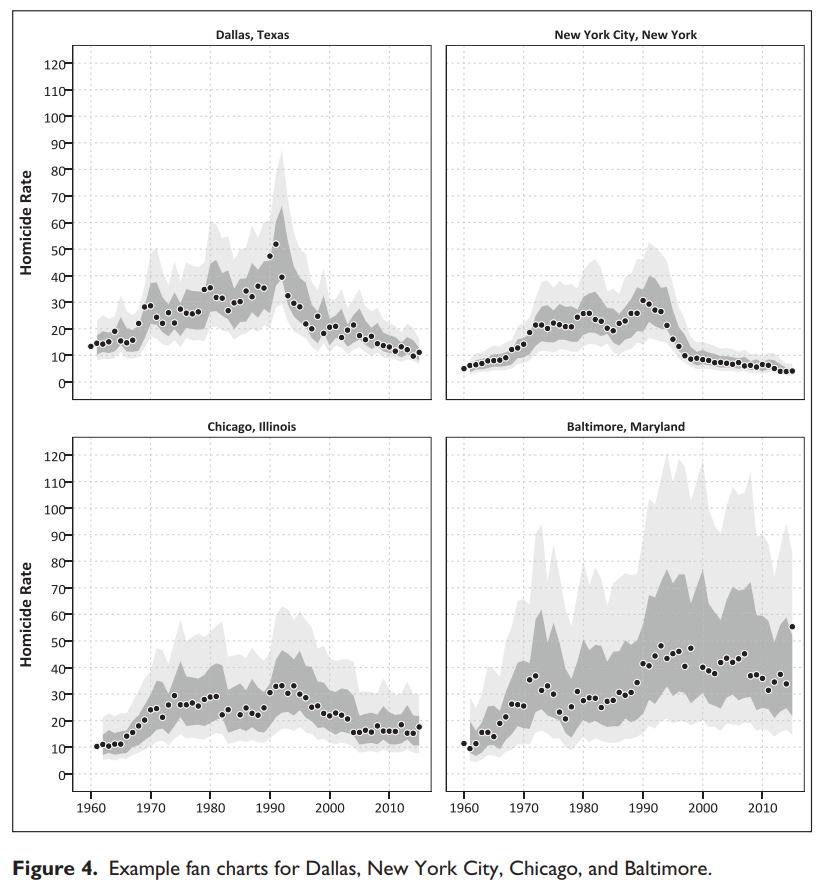As always if you have feedback or suggestions let me know! I posted all of the code to replicate the analysis at this link. The prediction intervals can definately be improved both in coverage and in making their length smaller, so I hope to see other researchers tackling this as well.